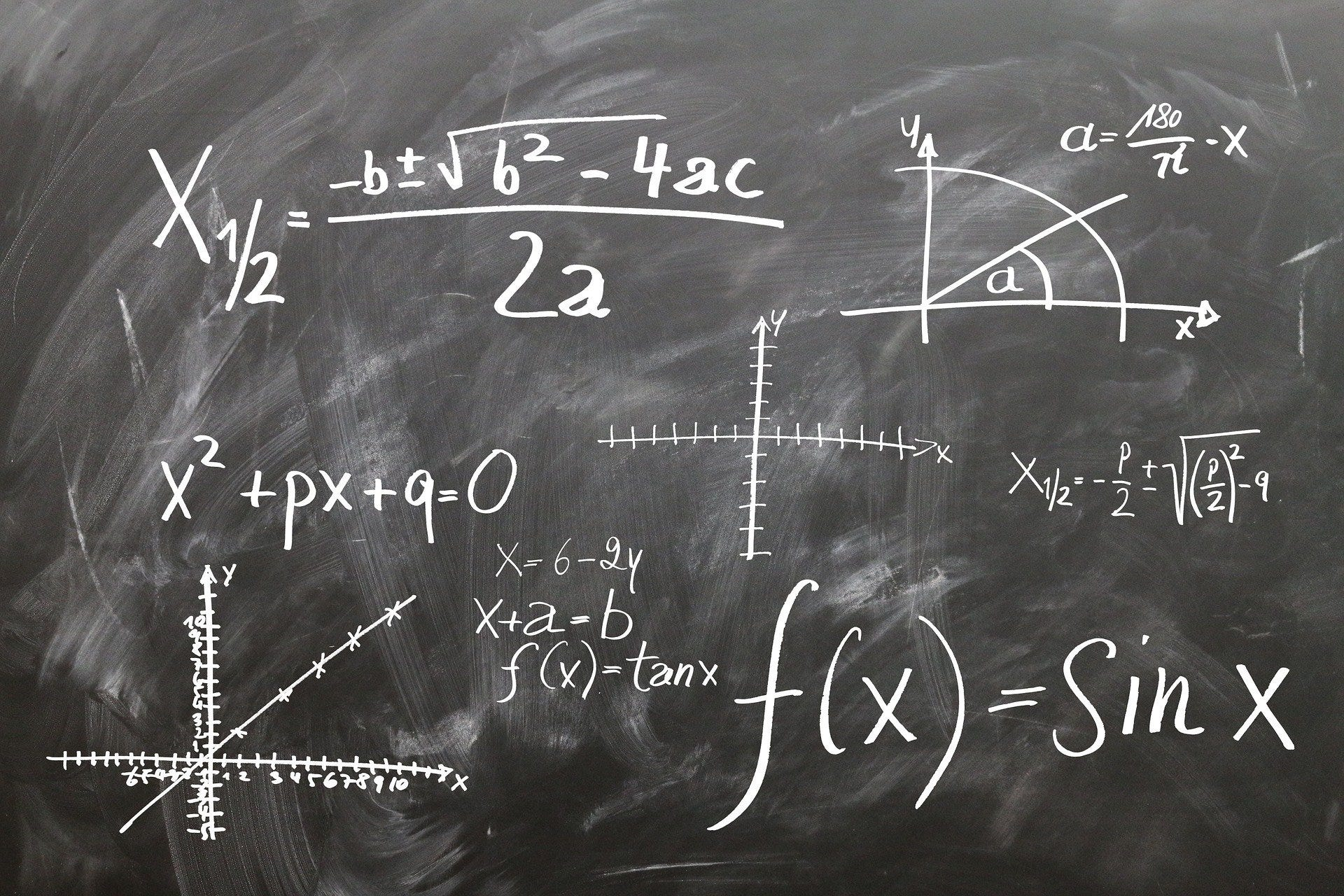Python‎ > ‎

### The Next Level of Functional Programming in Python

posted Nov 24, 2020, 7:31 AM by Chris G   [ updated Nov 24, 2020, 7:32 AM ]
From: https://towardsdatascience.com/the-next-level-of-functional-programming-in-python-bc534b9bdce1

## Useful Python tips and tricks to take your functional skills to the next levelImage by Gerd Altmann from Pixabay

preface: are you ready to take your Python skills to the next level? Can you walk through the examples in this story without squinting? Functional programming in Python might be daunting for some, but still, very fulfilling.

Python is one of the world’s most popular and in-demand programming languages. Indeed, if you are in the hot field of Data Science, Python is, most probably, your daily driver. But why?

• Python is easy to learn for beginners, even if they don’t have a Computer Science degree
• Python has a mature and supportive community and a huge range of modules and libraries
• Python is a versatile, extremely hackable, flexible, efficient and reliable programming language
• Python can help you automate menial tasks, such as creating, moving and renaming files or folders

However, this story is not for beginners. Some experience with the language is required to understand the examples and their value. Thus, without further ado let’s dive into the `itertools` module of Python, to discover a few hidden-in-plain-sight treasures of the language.

Learning Rate is a newsletter for those who are curious about the world of AI and MLOps. You’ll hear from me every Friday with updates and thoughts on the latest AI news and articles. Subscribe here!

# Definitions

Every function that we examine here is part of Python’s `itertools` library. That means that to import them you just write `from itertools import x`, where `x` is the function at hand. You will see that most of them, do not do anything useful by themselves, but the magic starts when you compose them.

Note that the examples work with Python `3.x`

• count: `count` takes a `start` and a `step` and generates all numbers from start onwards. For example:
`count(start=10, step=1) --> 10 11 12 13 14 ...`
• islice: `islice` returns a lazy slice out of a sequence. You can specify, where to start and where to stop, for example:
`islice('ABCDEFG', 2, None, 1) --> C D E F G`
• tee: `tee` splits an iterator `n` into two or more copies. Its definition is:
`tee(it, [n=2])`
• repeat: `repeat` simply repeats an element `n` times. If you omit `n` it will repeat the element forever. For example:
`repeat(elem=10, n=3) --> 10 10 10`
• cycle: `cycle` repeats the elements of a sequence over and over. For example:
`cycle('ABCD') --> A B C D A B C D ...`
• chain: `chain` takes sequences as input and goes over the elements of each sequence, one at a time. For example:
`chain('ABC', 'DEF') --> A B C D E F`
• accumulate: `accumulate` takes a sequence and a function, `add` by default, and generates the running totals. For example:
`accumulate(p=[1,2,3,4,5], func=add) --> 1 3 6 10 15`

As we said, to make something out of these primitive functions, we would have to compose them and create higher abstractions. So let’s do that and create our `itertools` extensions.

## Custom extensions

To begin with, let’s create a function to realize the first `n` elements of a sequence, for instance, a generator.

`def take(it, n):    return [x for x in islice(it, n)]`

As we do that, let’s also create a function that removes the first `n` elements of a sequence.

`def drop(it, n):    return islice(it, n, None)`

Now, we are able to create our own primitive functions, that return the `head` and `tail` of a sequence.

`head = nexttail = partial(drop, n=1)`

If you haven’t use `partial` before, you can find it in the `functools` namespace. In this example, it takes the drop function, passes `1` as the argument `n`, and returns a new function that expects the `it` argument. Thus, if you pass a list in the `tail` function, it chops off the first element and it returns the remaining items.

Next, we want to create a new function, that we will call compose. It works like that:

`compose(x, f) --> x, f(x), f(f(x)), f(f(f(x))), ... `

So, how can we do that? It turns out we can do this very efficiently now that we have yielded the power of the `repeat` and `accumulate` functions:

`def compose(x, f):    return accumulate(repeat(x), lambda acc, x: f(acc))`

I admit it looks a bit complex, but if you squint hard enough, you’ll understand its inner workings. `repeat` generates an infinite sequence of `x`s and then, `accumulate` takes each of them and calculates the running total according to some function `f` that we define. So, the `lambda` function, takes as inputs the previously accumulated value, and a new `x` that we ignore, and passes the accumulated value through the function, again and again. To test it, we could do something like that:

`take(compose(2, lambda x: x**2), 5) --> [2, 4, 16, 256, 65536]`

# Putting it all together

Now, we are onto something here! So let’s use some functions that we have defined to create the infamous Fibonacci numbers sequence.

`def next_num(pair):    x, y = pair    return y, x + ydef fibonacci():    return (y for x, y in compose((0, 1), next_num))`Down the Fibonacci hole — Photo by Ludde Lorentz on Unsplash

Let’s walk it through step by step. The compose function creates a sequence of tuples. The first tuple is `(0, 1)`. Thus, the `fibonacci` function yields the `y` value of the tuple, which is `1`. It then pass the tuple `(0, 1)` to the `next_num` function, which returns `1` and `0 + 1 = 1`. So, the `fibonacci` function yields also `1`. Finally, the `compose` function passes the tuple `(1, 1)` to the `next_num` function, which returns `1` and `1 + 1 = 2`. The `fibonacci` function in turn yields `2`.

`take(fibonacci(), 10) --> [1, 1, 2, 3, 5, 8, 13, 21, 34, 55]`

This is a super-efficient implementation of the Fibonacci sequence, which runs under 35μs! It showcases the power of good, functional design!